• Weather
• Ocean
• Ice & Icebergs
• Other Hazards
• Archive Data
• Digital Forecasts
• Electronic Marine Charting (ECDIS)
• Environmental
• Extratropical Storm Surge Models
• Freezing Spray
• GIS
• Observations
• Ocean
• Probabilistic
• Product Information
• Receiving Products
• About OPC
• News
• Social Media

## GEFSWAVE Probabilistic Wave Height Guidance

Use the drop down menus below to change the display.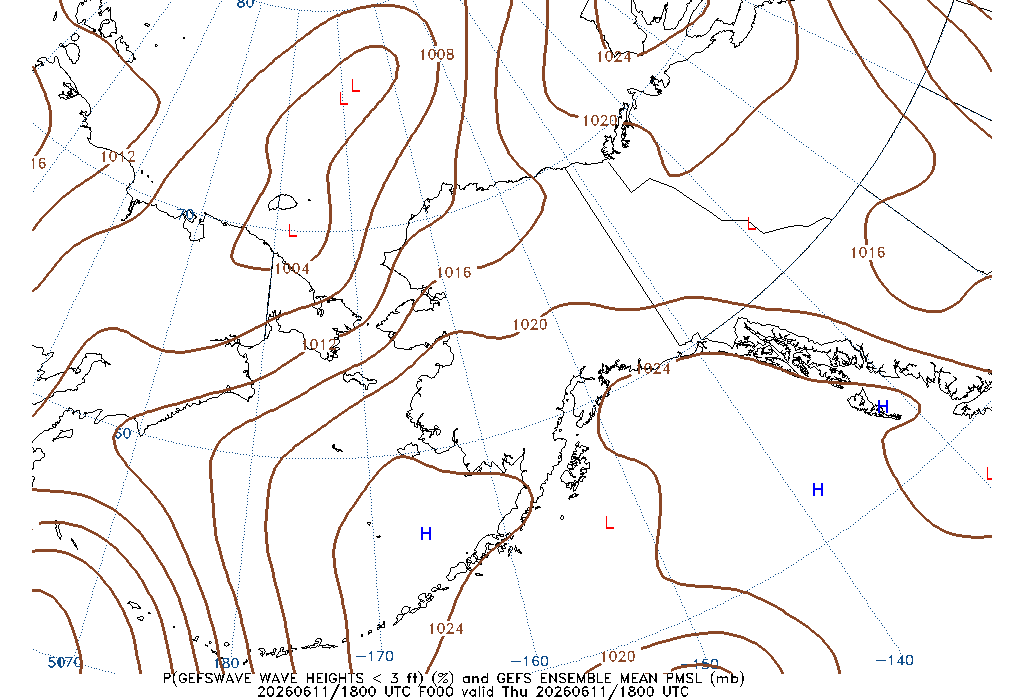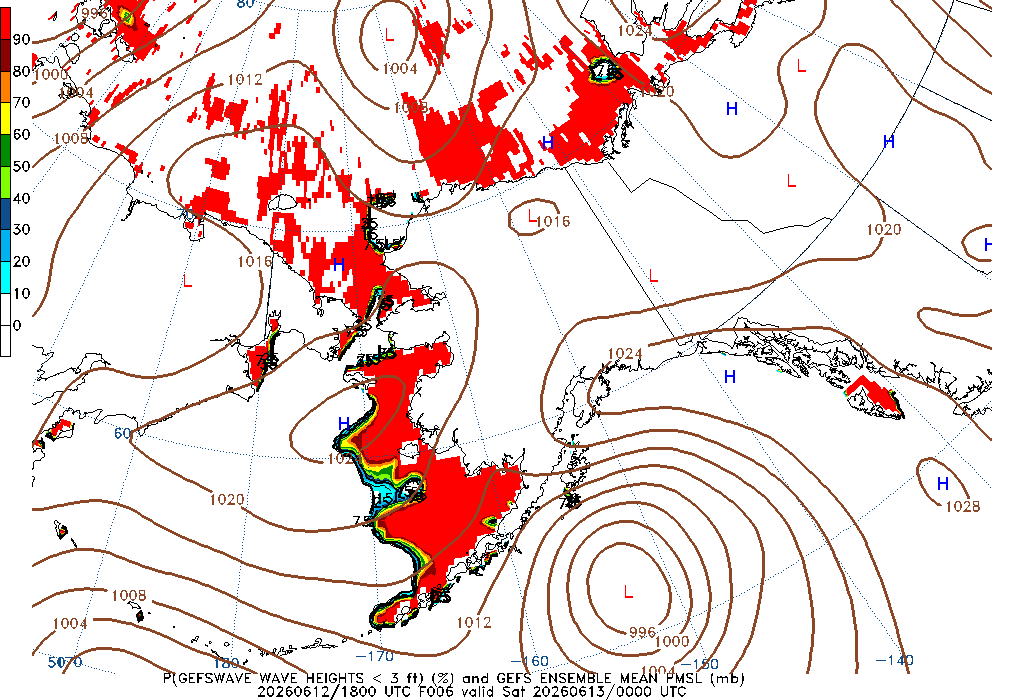000 Hour Wave Height less than 3ft 006 Hour Wave Height less than 3ft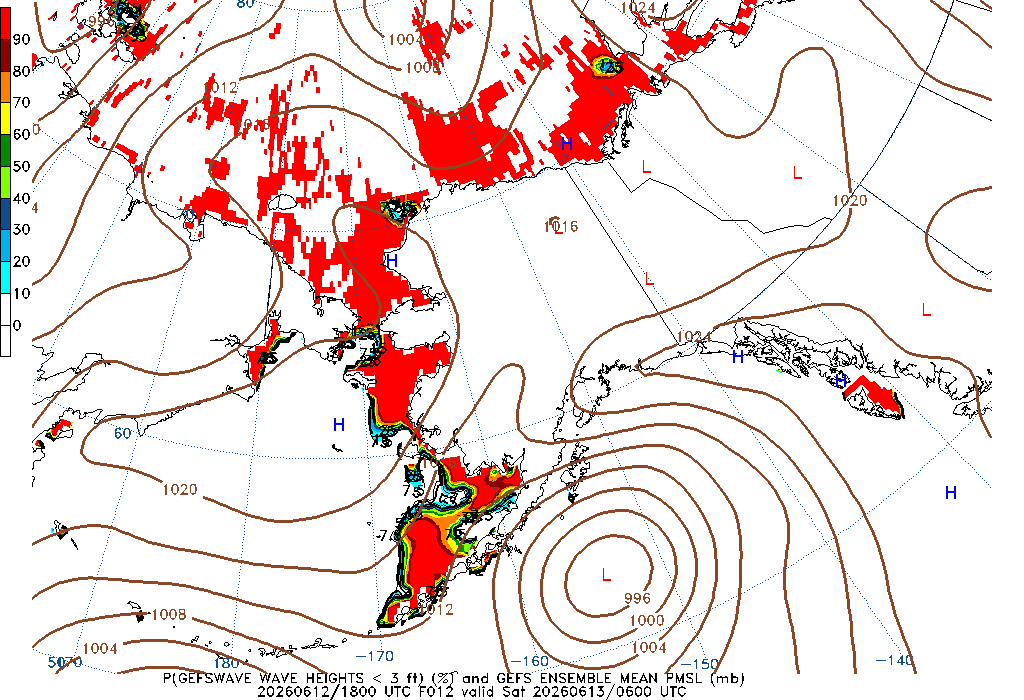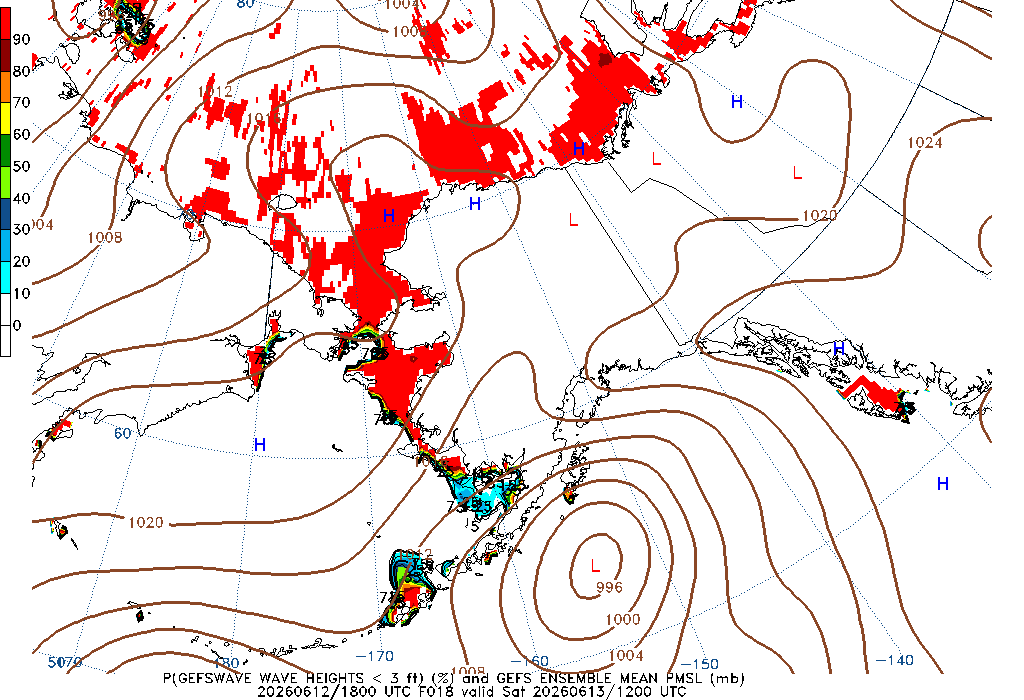012 Hour Wave Height less than 3ft 018 Hour Wave Height less than 3ft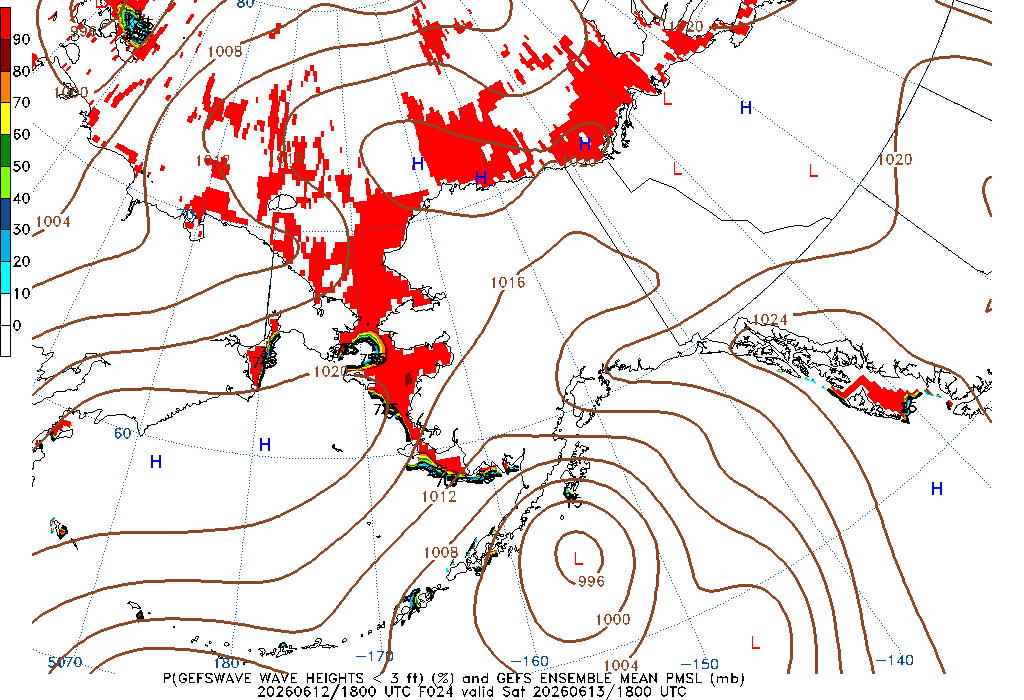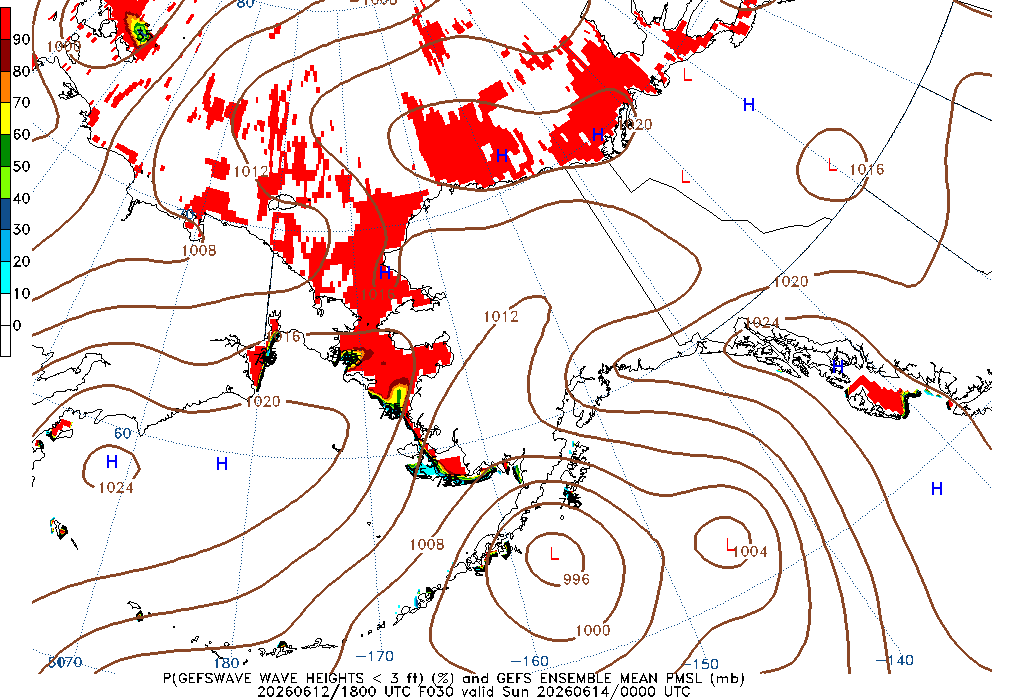024 Hour Wave Height less than 3ft 030 Hour Wave Height less than 3ft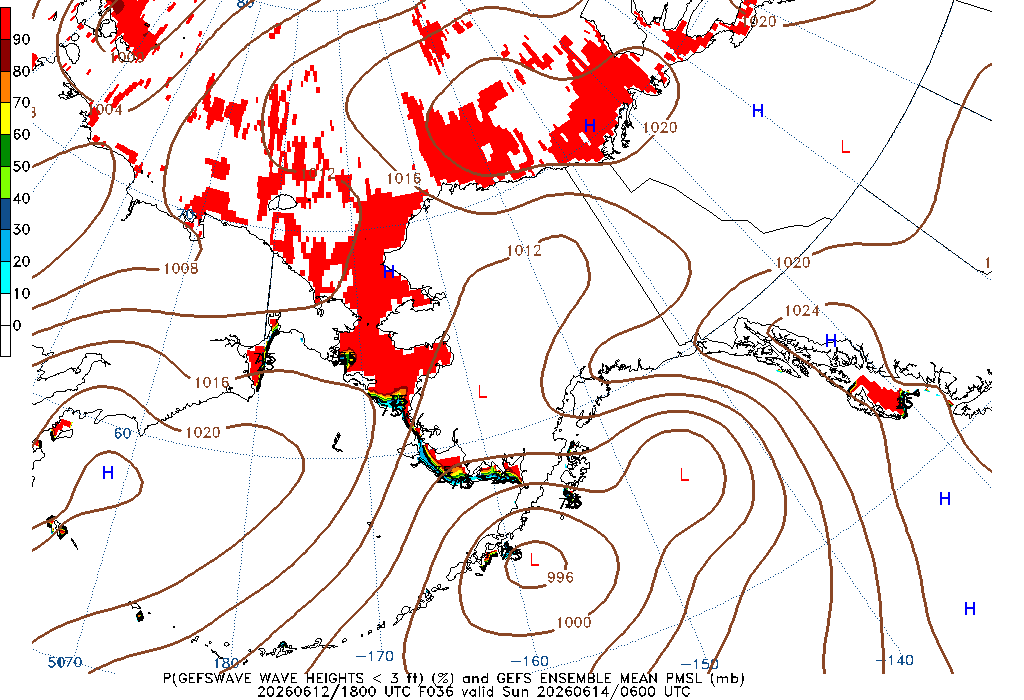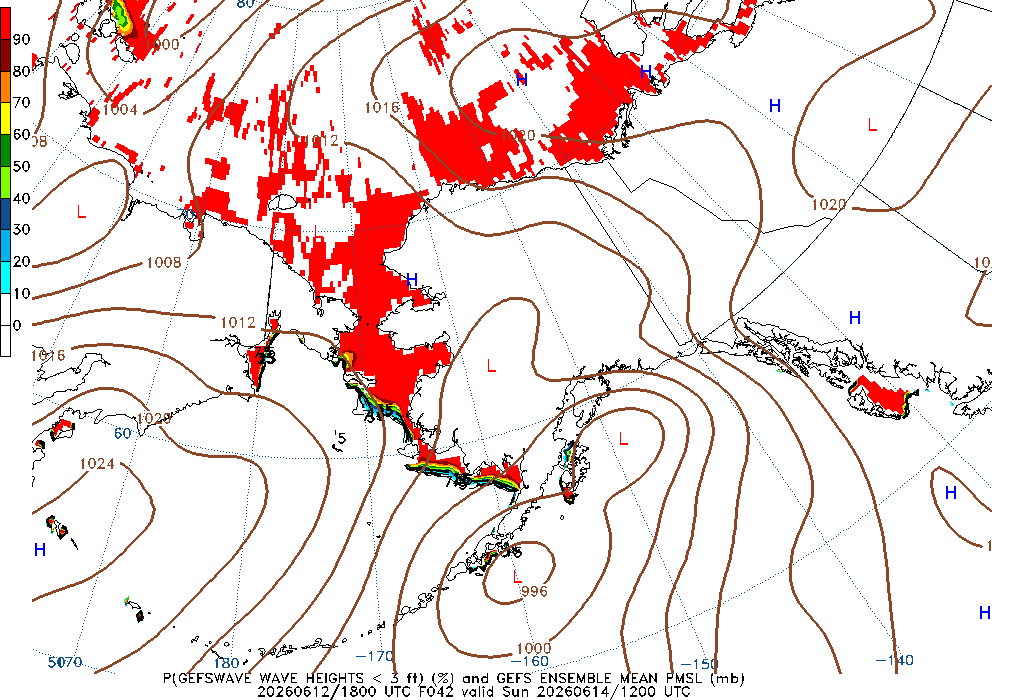036 Hour Wave Height less than 3ft 042 Hour Wave Height less than 3ft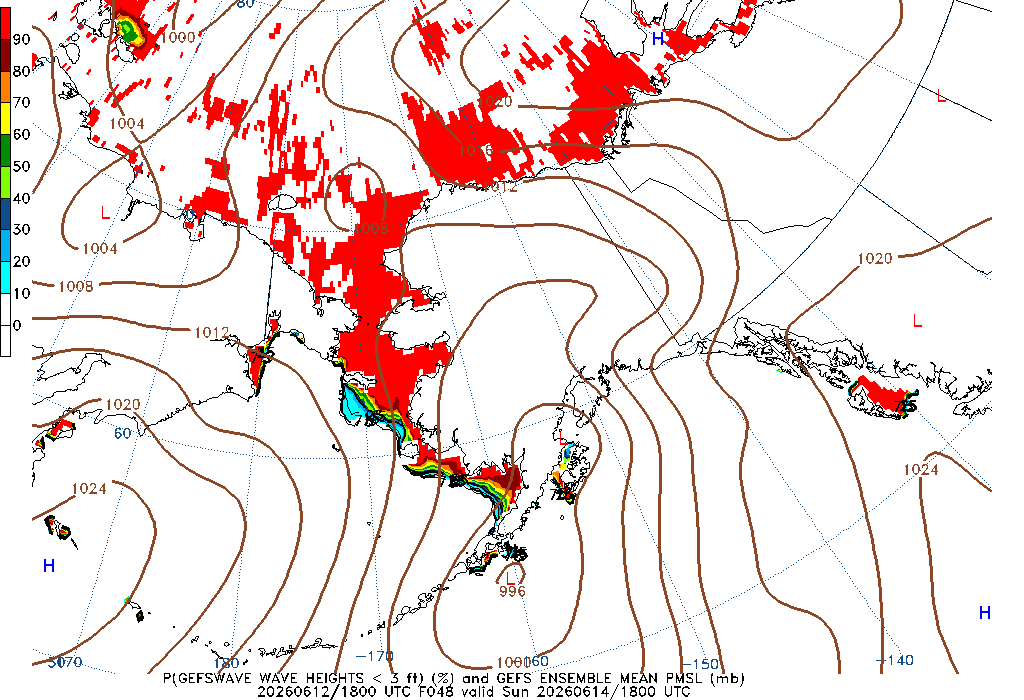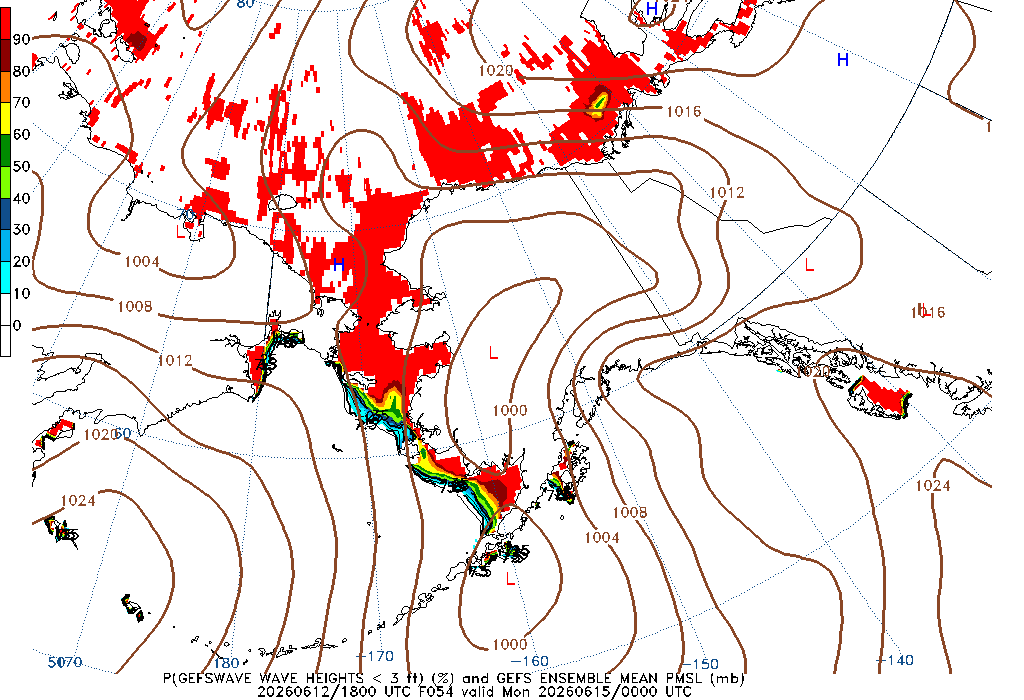048 Hour Wave Height less than 3ft 054 Hour Wave Height less than 3ft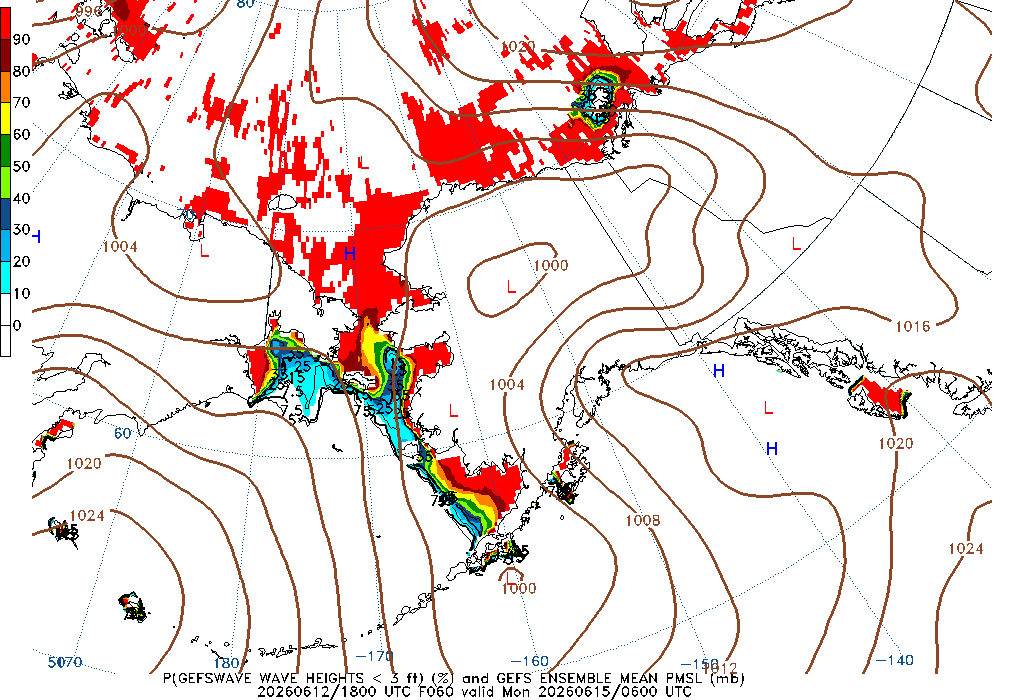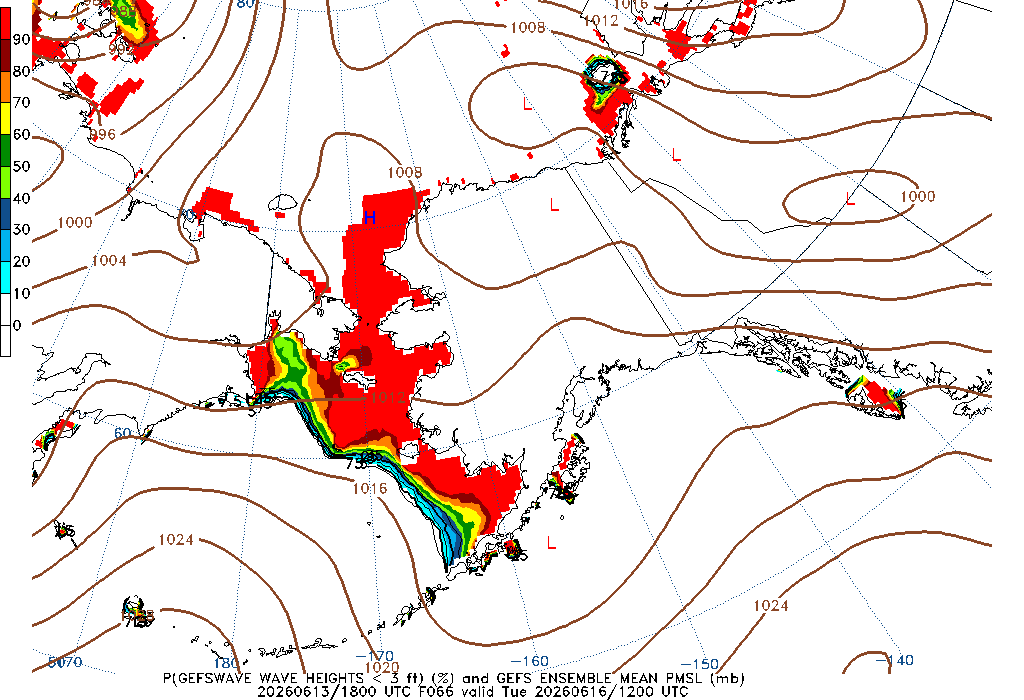060 Hour Wave Height less than 3ft 066 Hour Wave Height less than 3ft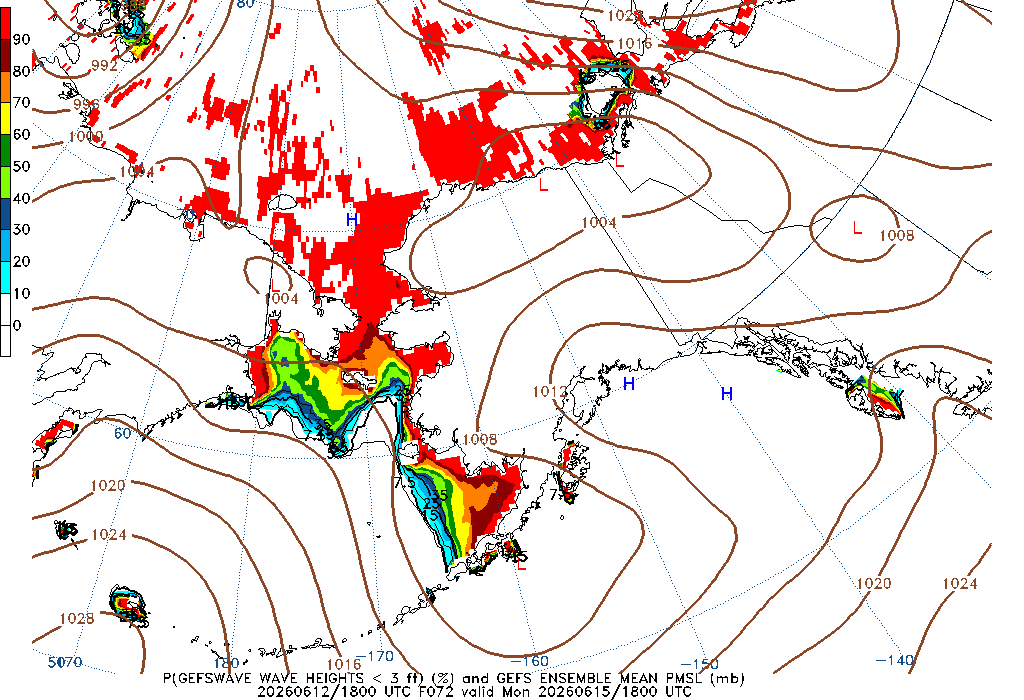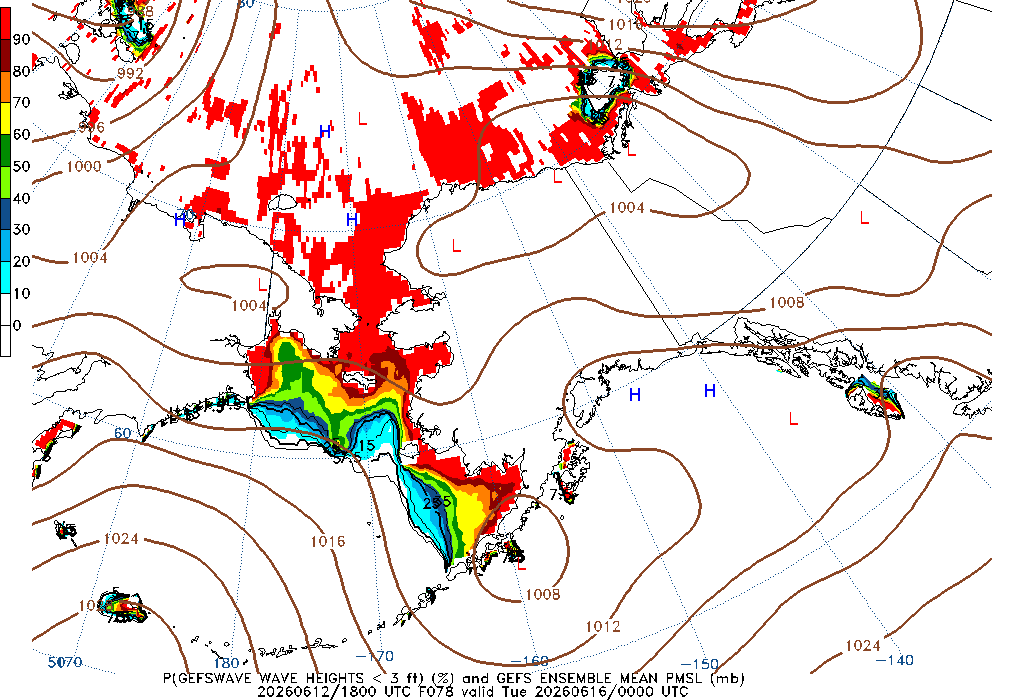072 Hour Wave Height less than 3ft 078 Hour Wave Height less than 3ft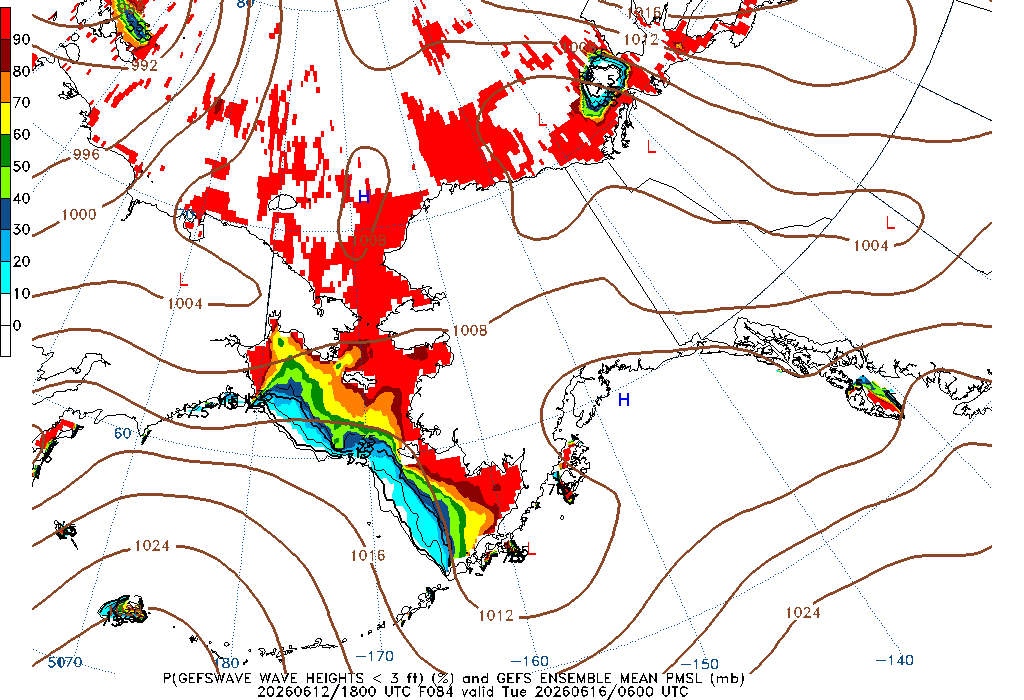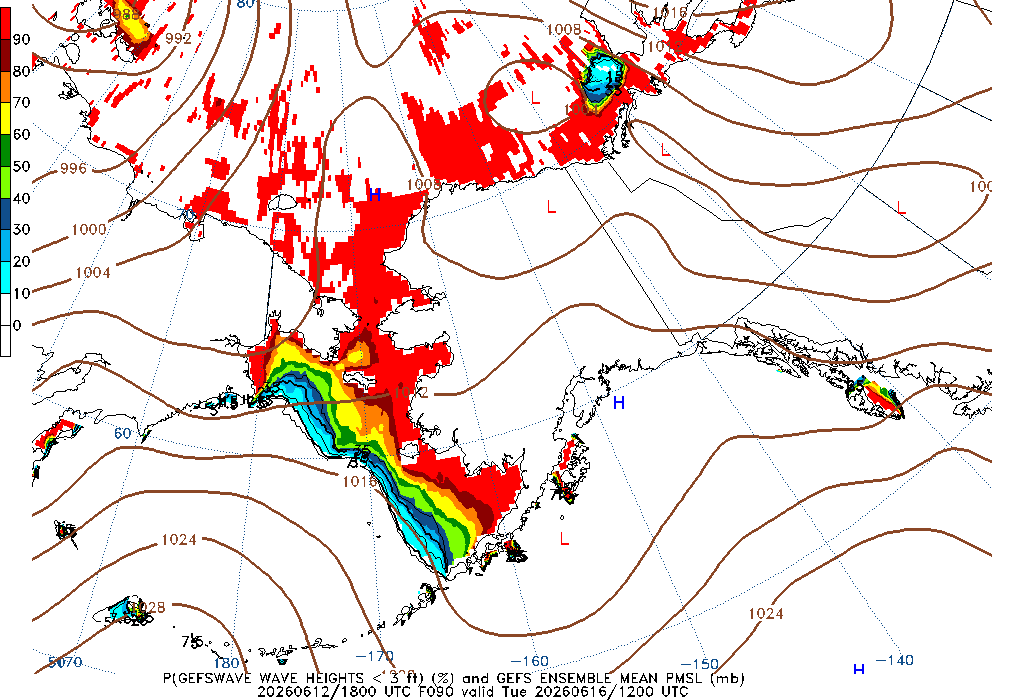084 Hour Wave Height less than 3ft 090 Hour Wave Height less than 3ft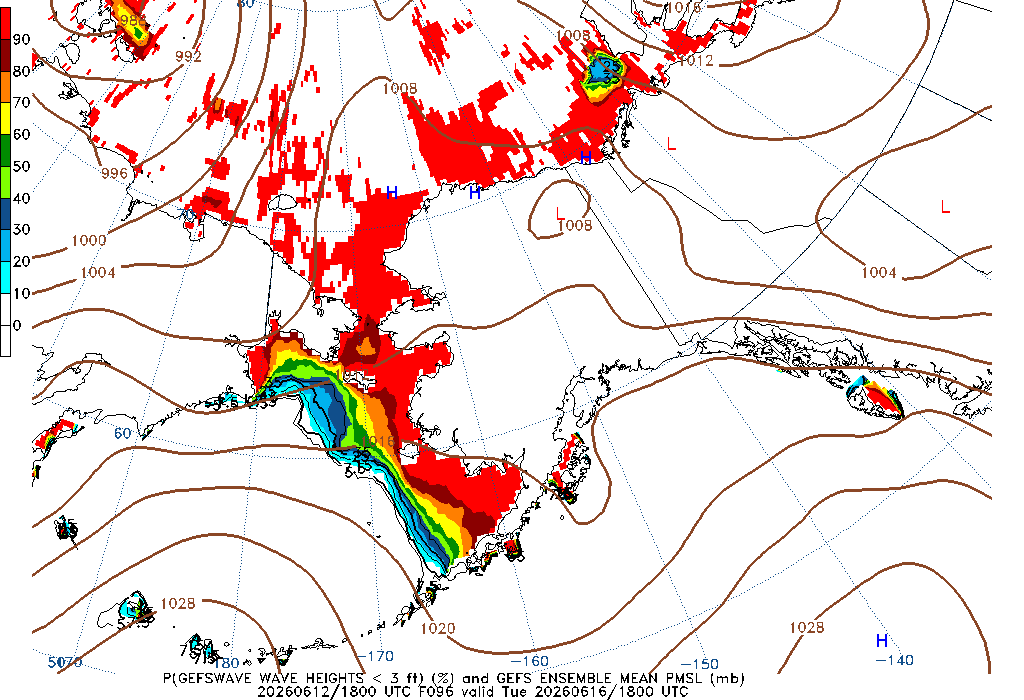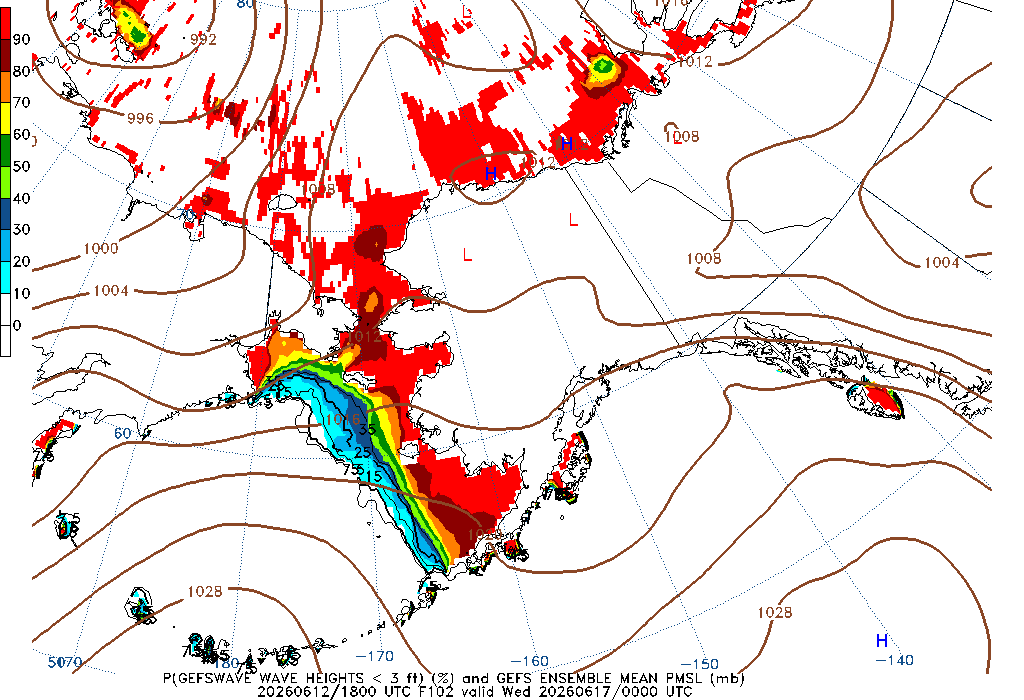096 Hour Wave Height less than 3ft 102 Hour Wave Height less than 3ft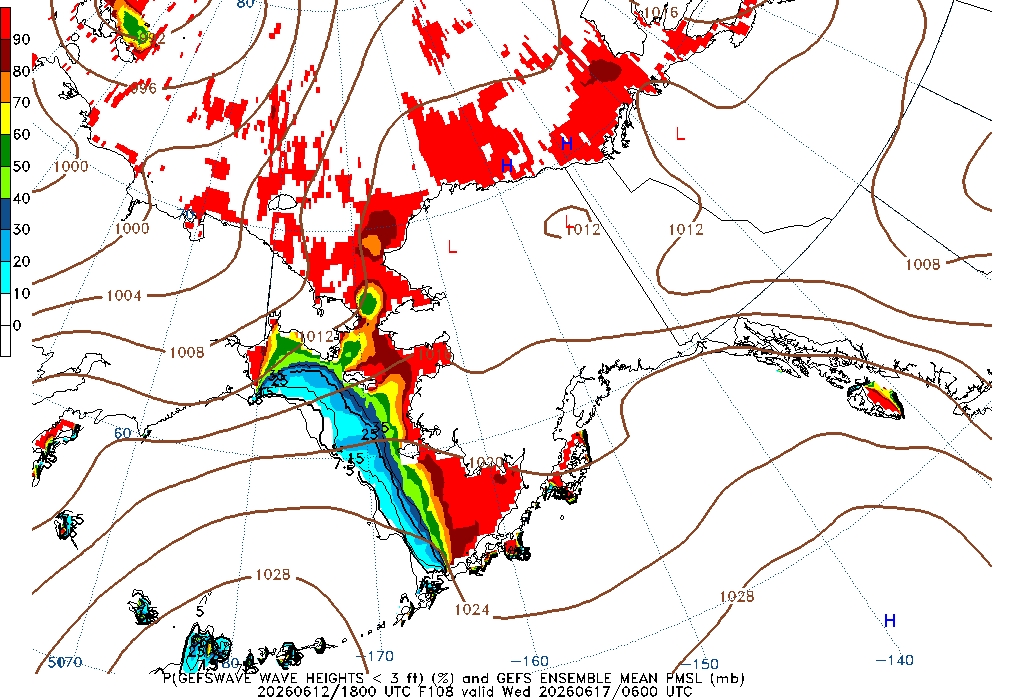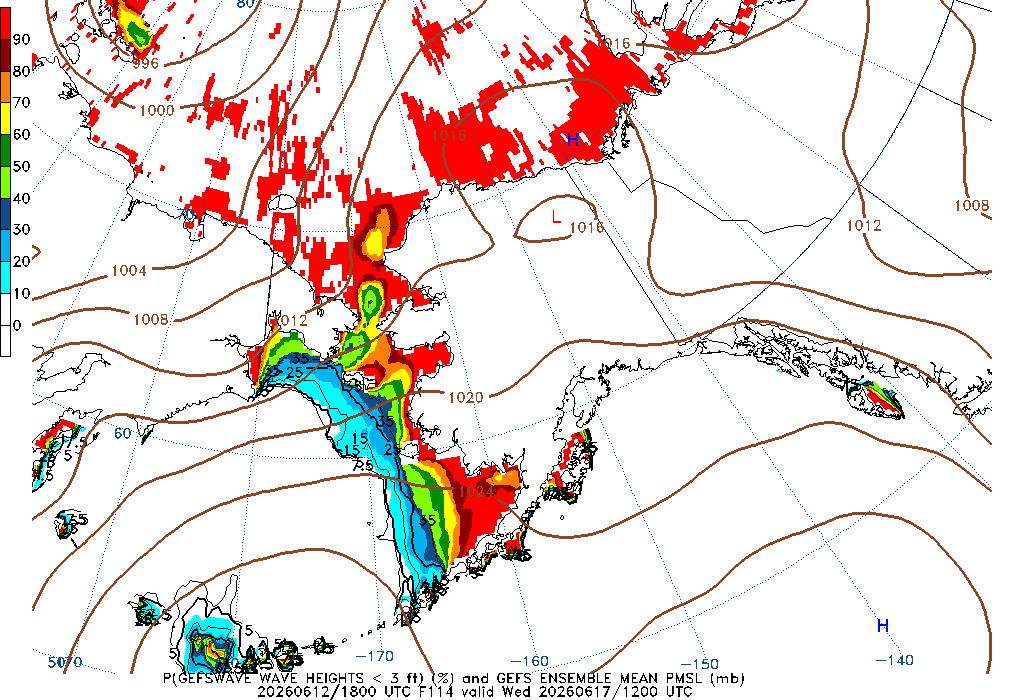108 Hour Wave Height less than 3ft 114 Hour Wave Height less than 3ft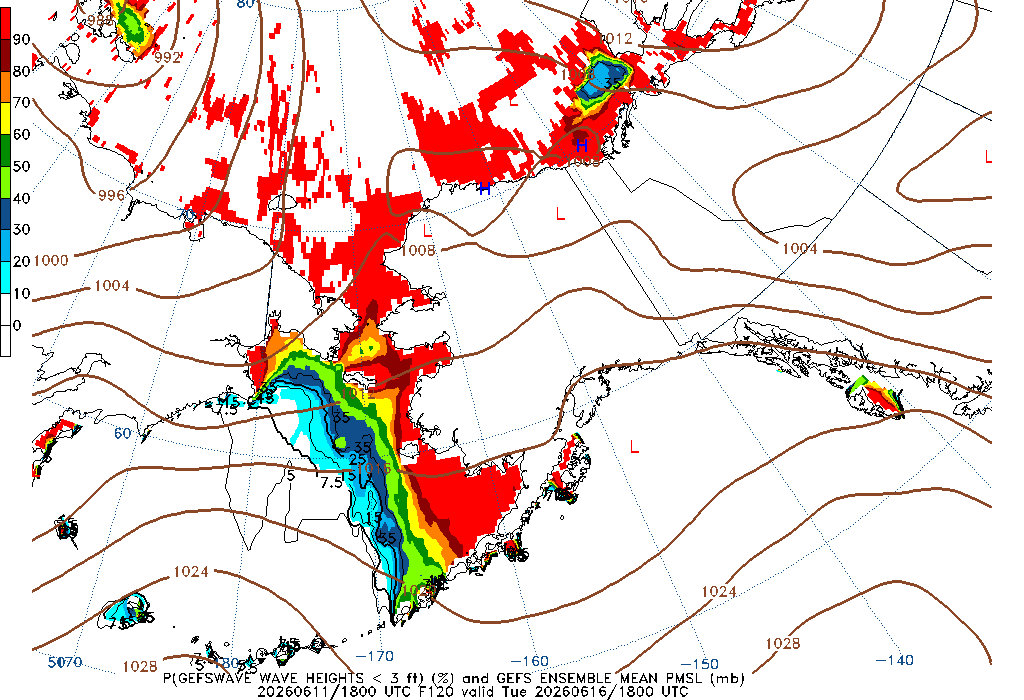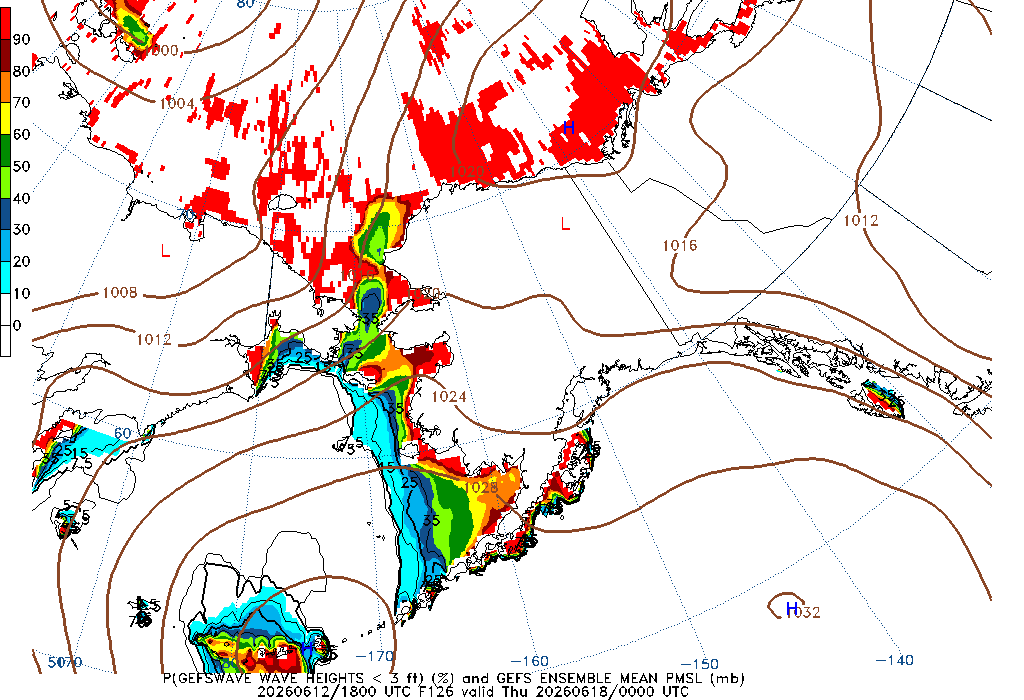120 Hour Wave Height less than 3ft 126 Hour Wave Height less than 3ft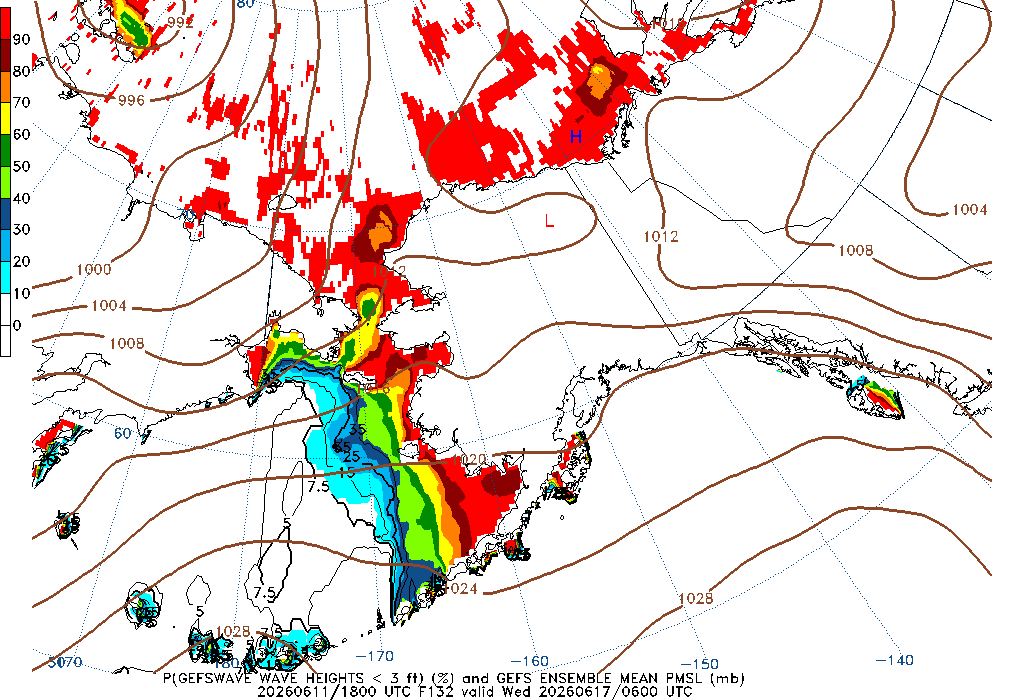132 Hour Wave Height less than 3ft 138 Hour Wave Height less than 3ft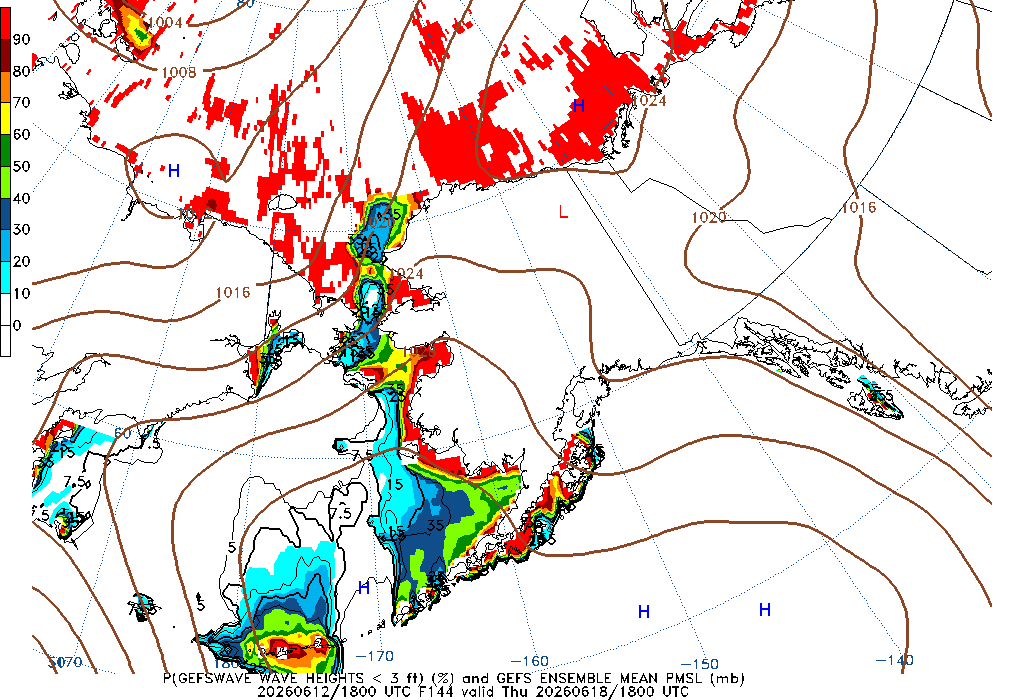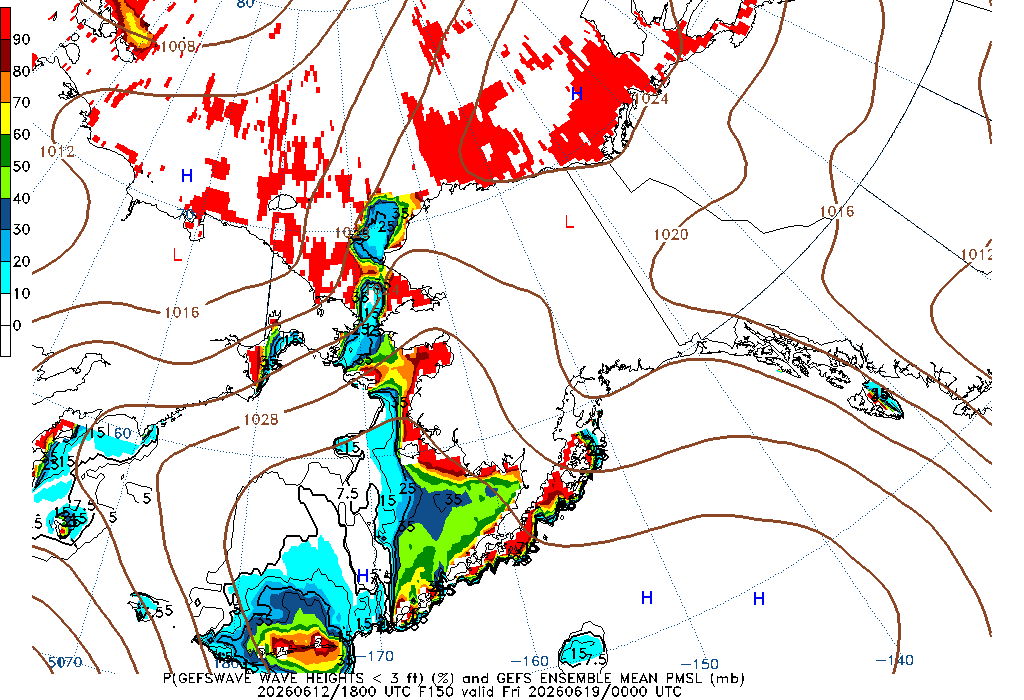144 Hour Wave Height less than 3ft 150 Hour Wave Height less than 3ft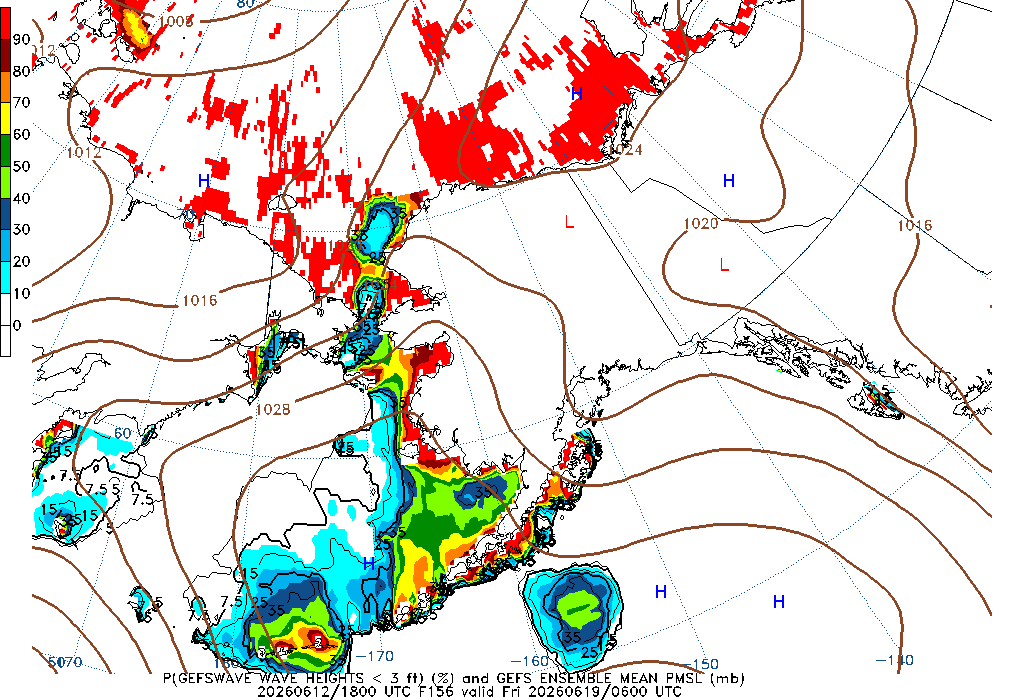156 Hour Wave Height less than 3ft 162 Hour Wave Height less than 3ft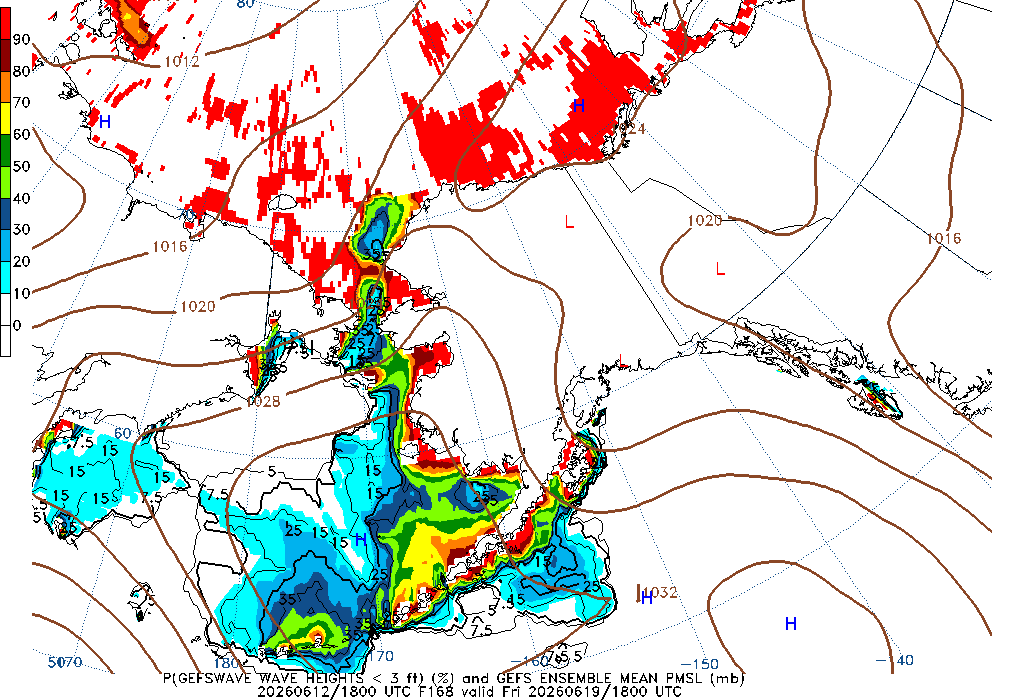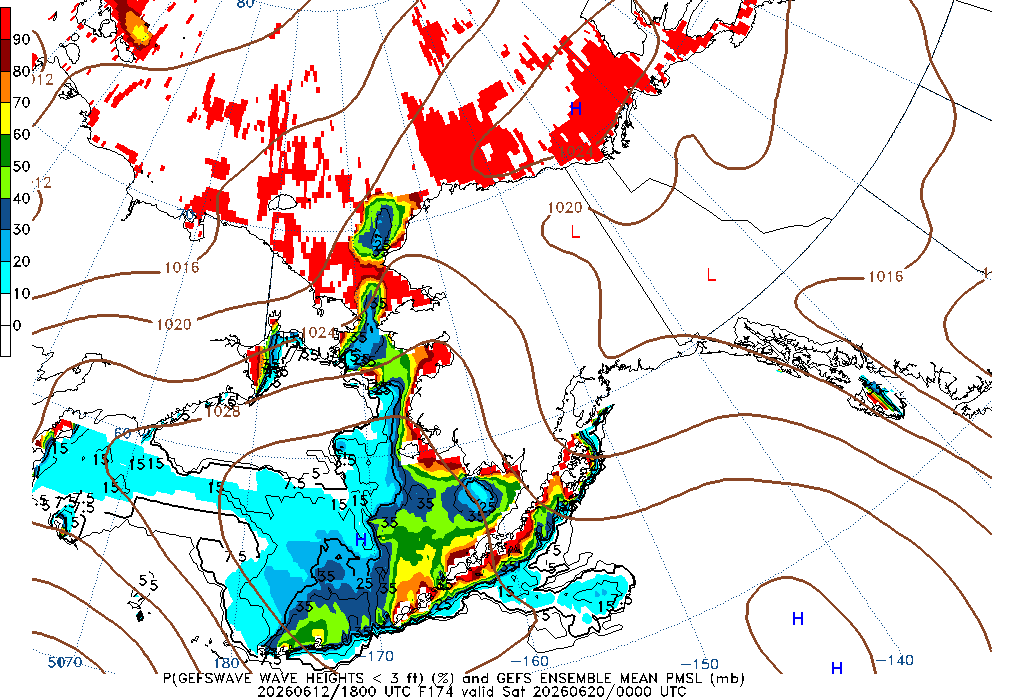168 Hour Wave Height less than 3ft 174 Hour Wave Height less than 3ft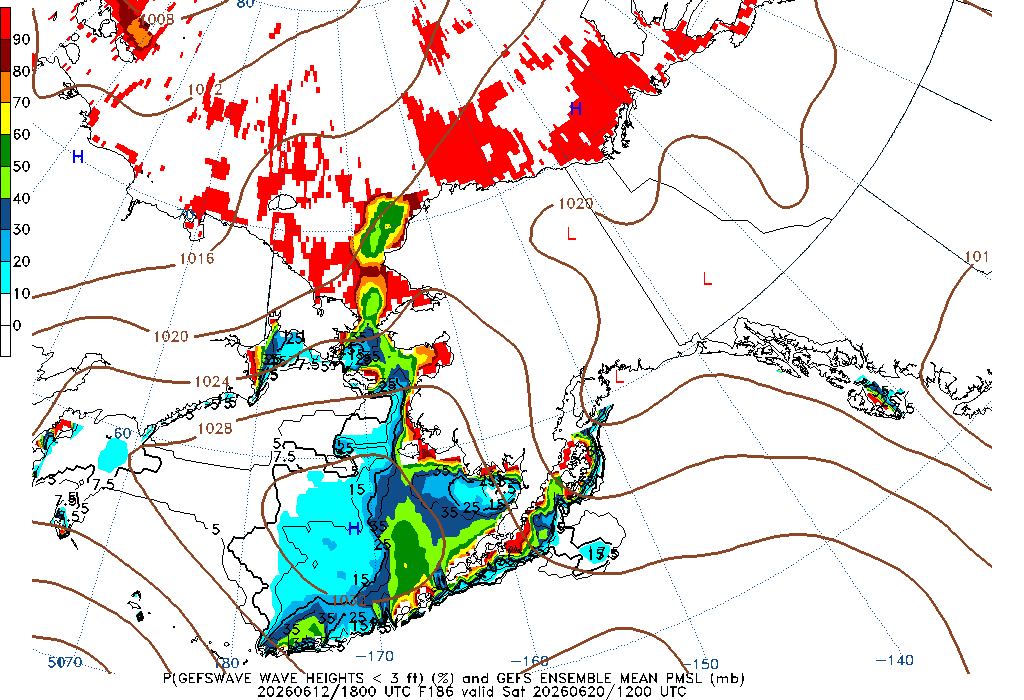180 Hour Wave Height less than 3ft 186 Hour Wave Height less than 3ft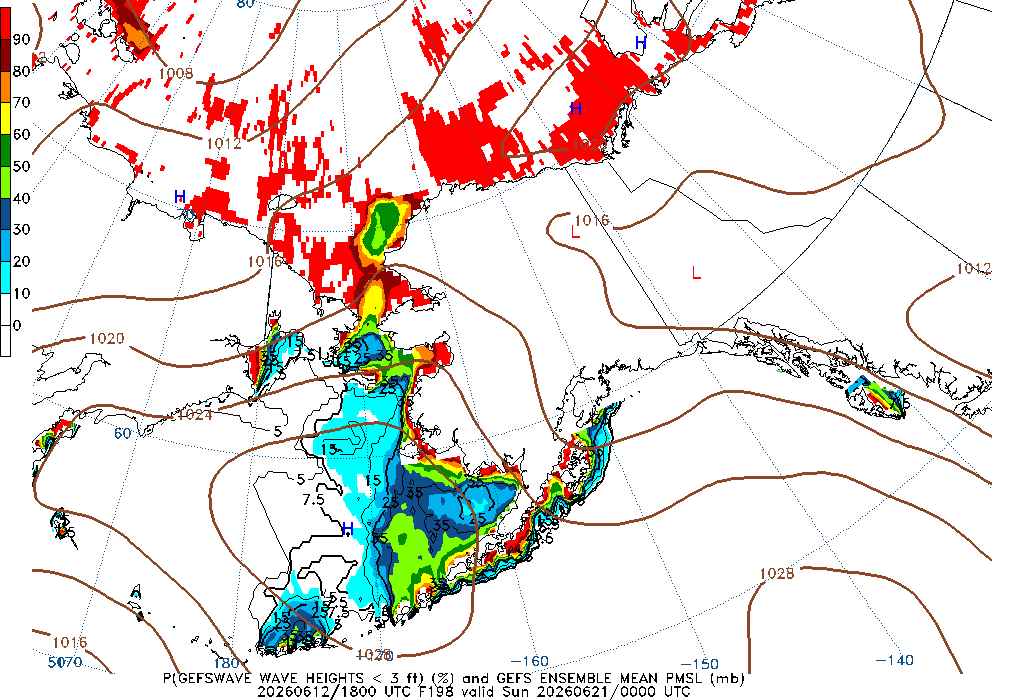192 Hour Wave Height less than 3ft 198 Hour Wave Height less than 3ft204 Hour Wave Height less than 3ft 210 Hour Wave Height less than 3ft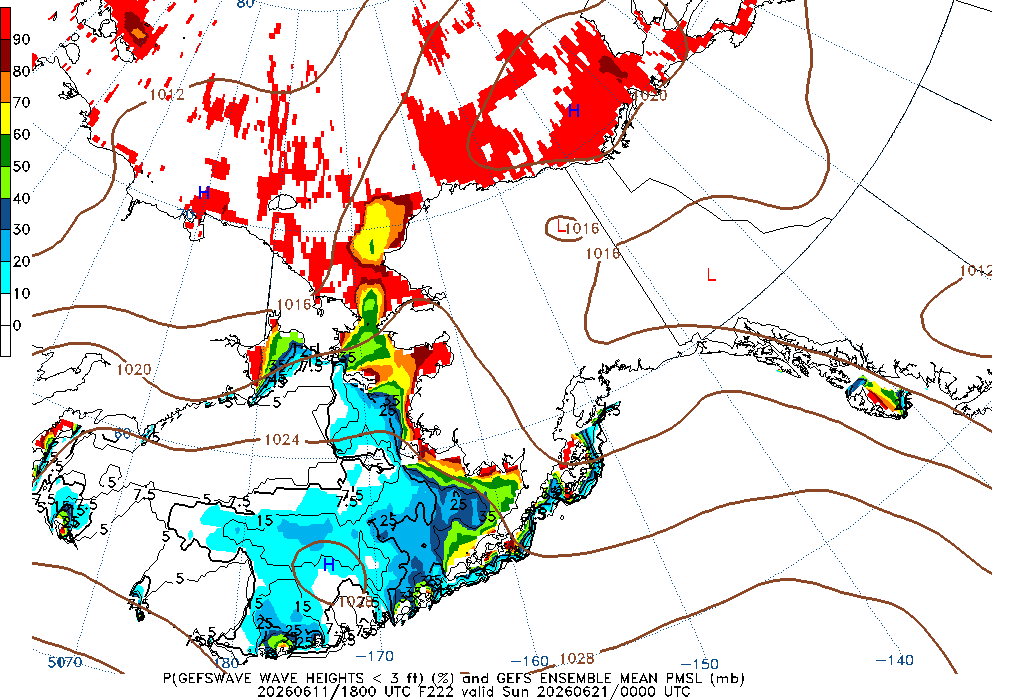216 Hour Wave Height less than 3ft 222 Hour Wave Height less than 3ft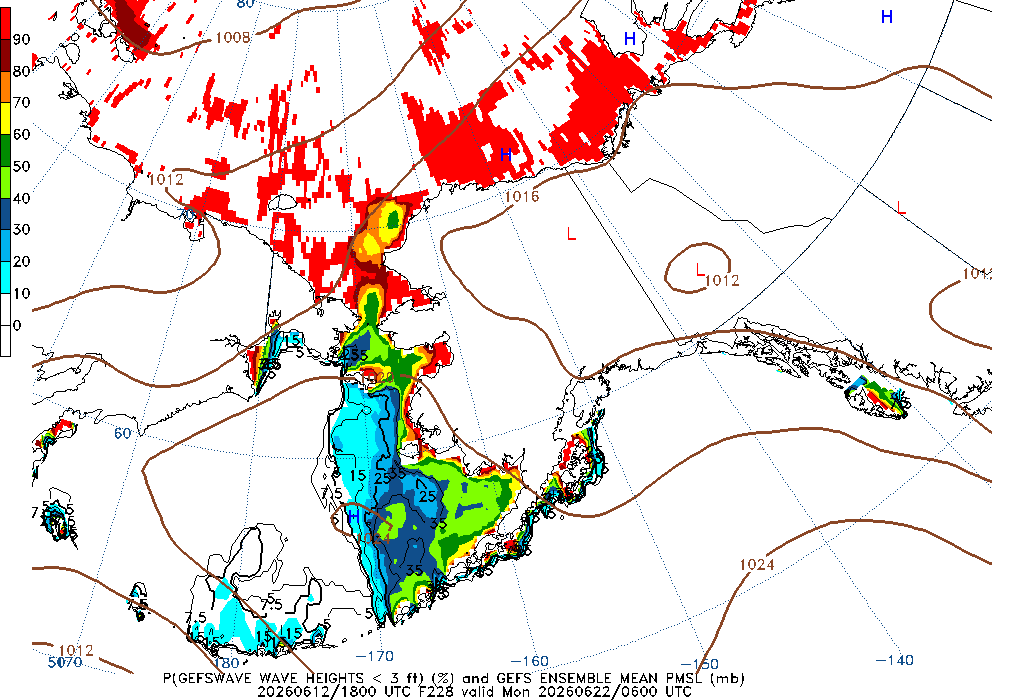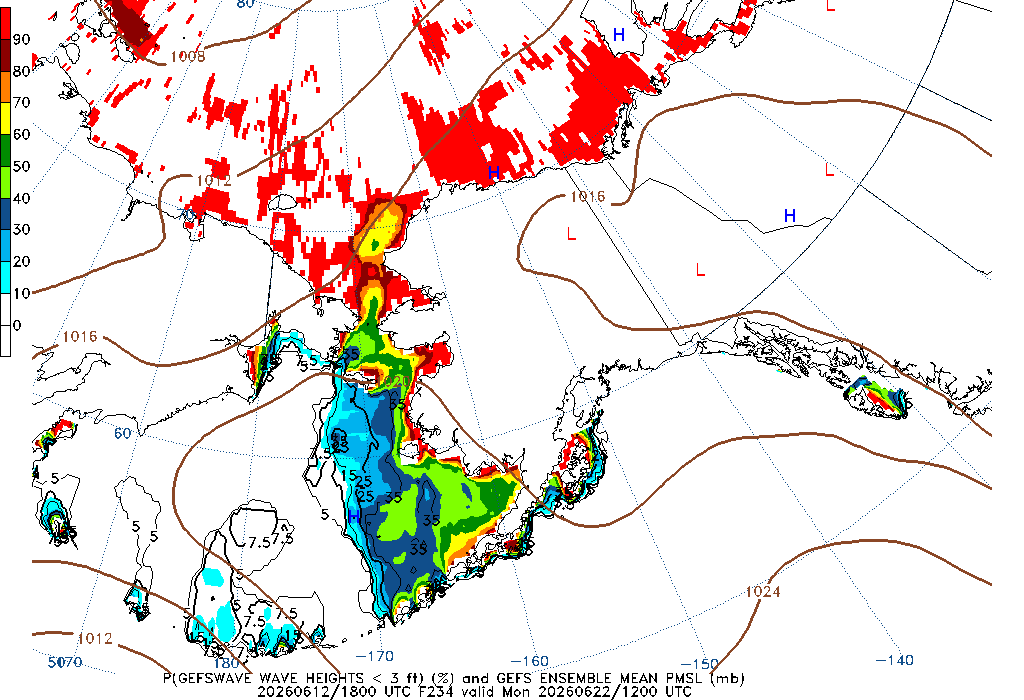228 Hour Wave Height less than 3ft 234 Hour Wave Height less than 3ft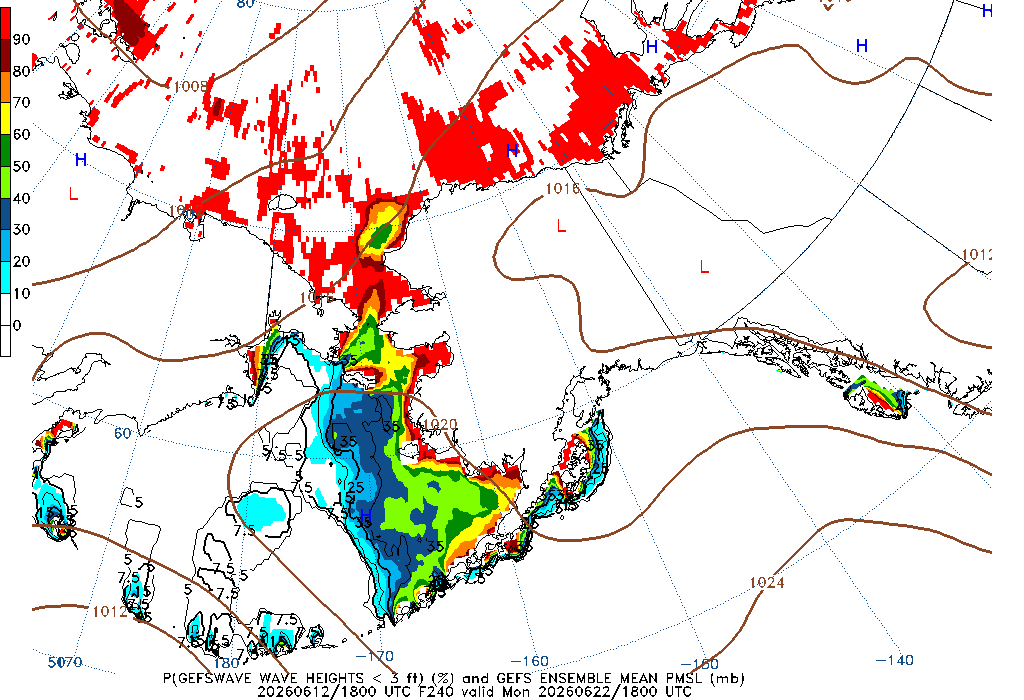240 Hour Wave Height less than 3ft

Notes on images above:

• The likelihood of exceedance is shown as a probability of 0 to 100 of waves heights (significant wave height) reaching or being greater than the chosen value.

• The 90th percentile represents the wave height value that 90% of the ensemble members are either less than or equal to (i.e., 18 feet).

• The 10th percentile is the wave height value that is represented by 10% of the contributing ensemble members.

• The mean is defined as the 50th percentile, that is, the wave height value represented reflects half the distribution is above and half is below this value.

• The maximum value is the highest value produced by any of the 31 ensemble members. It reflects the most severe conditions predicted by the ensemble prediction system.

The data are produced from the Global Ensemble Forecast System (GEFS) which is run every 6 hours and are created with 31 ensemble members.

Additional information for interpreting this data

More information is available from NOAA's Environmental Modeling Center (EMC).